# How to Use the SUM Function in Excel

Joshua is a graduate student at the USF. He has interests in business technology, analytics, finance, and lean six sigma.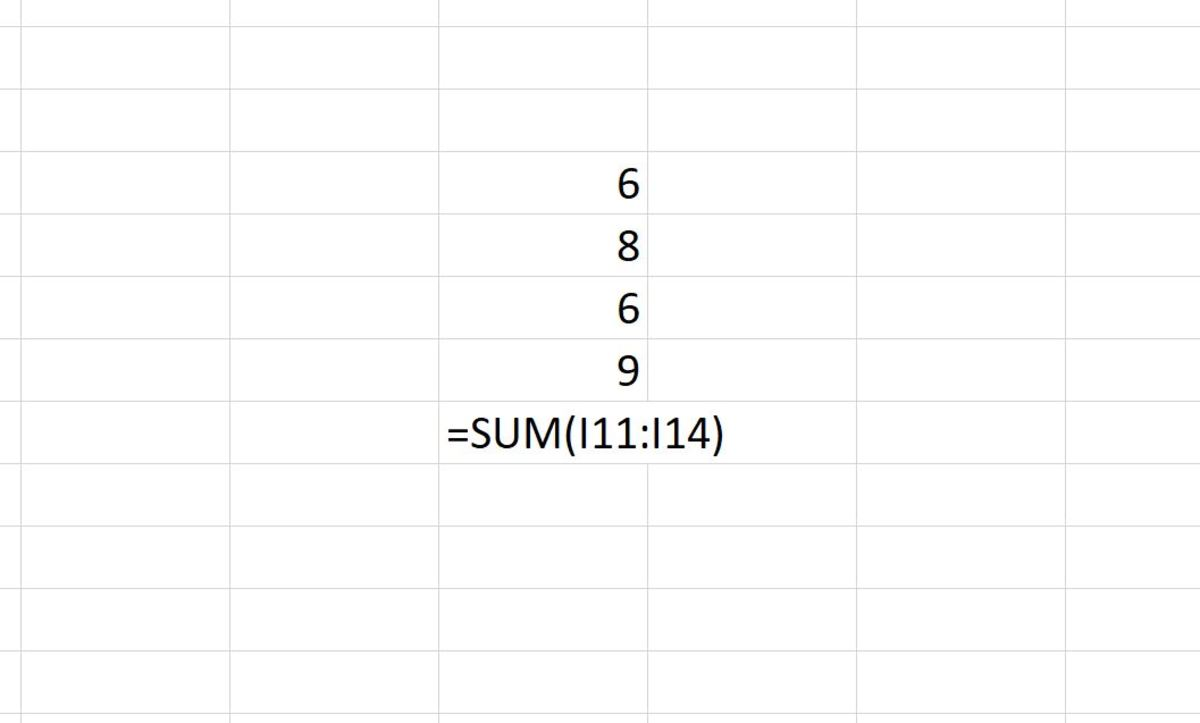The SUM function is shown in the above illustration summing a range of four cells with the first cell of the range followed by a colon and the last cell of the range. Created by Joshua Crowder

## The Purpose of the SUM Function

The SUM function is used to add values together from different cells in a worksheet or add numbers directly in a formula. This may be one of the most widely used functions in the history of Excel. Using the SUM function has proven to be a shortcut to adding numbers to a worksheet.

In this article, you will find out how the SUM function is used as well as the many ways it can be practically applied in Excel. Additionally, you will see how to add the function to Excel using four different methods.

## The SUM Function Syntax

The syntax for the SUM function is shown here:

=SUM(number1,[number2],[number3],[number4]...)

The SUM function is very straightforward. Numbers and cell references can be used to get a sum of multiple numbers. A range is usually used as a reference to collect data for this function. The function needs to be inputted like a formula to work properly. To manually add this formula to a cell, the following steps need to be followed:

1. A cell needs clicked and =SUM( needs to be typed.
2. After the open parenthesis, data is referenced are entered (numbers, a range, named ranges, a cell reference).
3. A closing parenthesis is used to close the formula.
4. The enter button can be pressed to return the sum of the data.

## Sum a Range of Data

You would sum a range like in the illustration below when all of the data that needs to be added together can be found in the same area of your spreadsheet.

Data can be selected vertically or horizontally with multiple rows and columns. An example of this technique can be seen in the illustration below.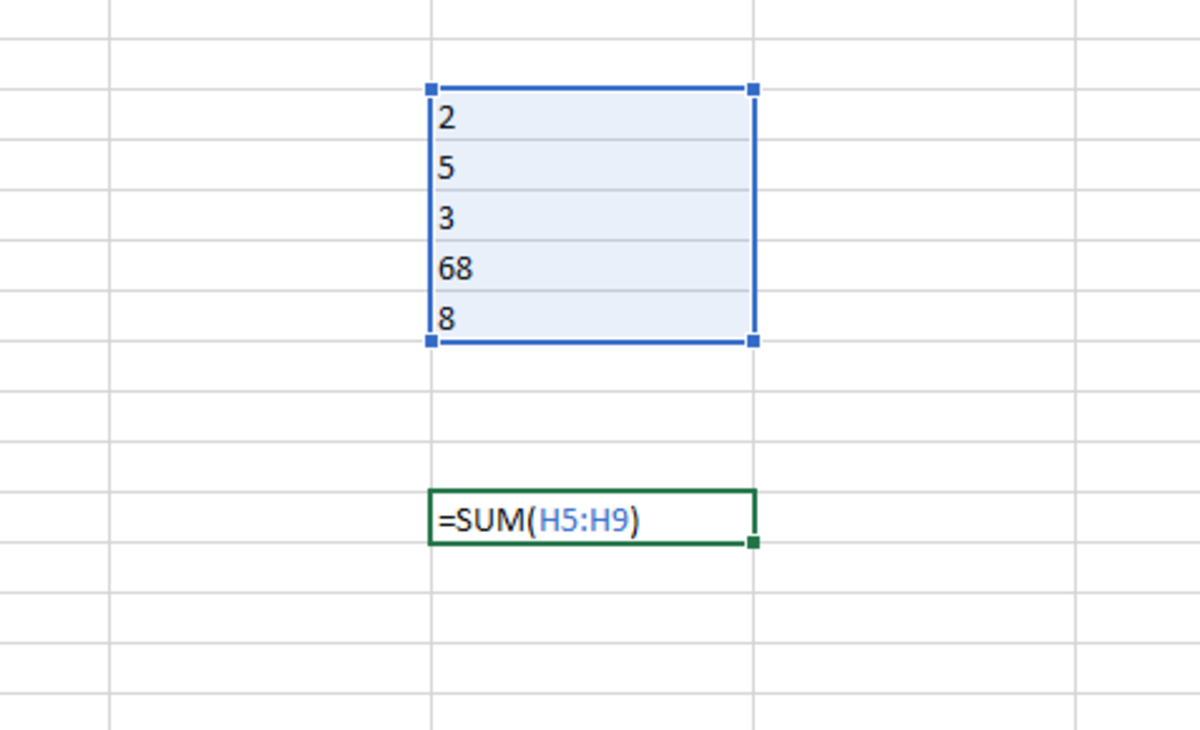In the above illustration, the SUM function is used to sum numbers that are aligned in a vertical range. The position of the range is represented by the first cell followed by a colon and the last cell of the range. Created by Joshua Crowder

## Sum With Cell References

You may opt to sum using cell references. This will allow you to sum from a vast number of cells from different areas of a workbook.

Each reference needs to be added to the formula and separated by a comma. This technique can be seen as being used in the illustration below.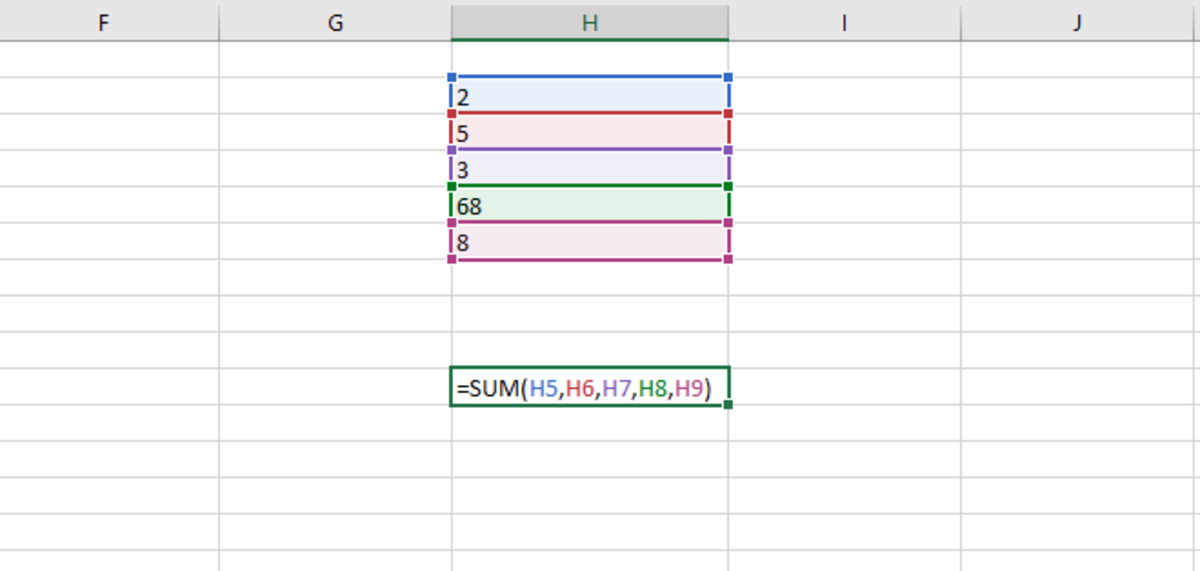In the above illustration, the SUM function is used to sum numbers with references to individual cells. This is done by adding each reference to the function and separating them by commas. Created by Joshua Crowder

## Sum Using Numbers

You can also sum with just numbers alone without using any ranges or references. This might be useful when a set of numbers always stays the same, and you want to add a cell reference as a variable to the set of numbers.

An example of using numbers only in the SUM function can be seen in the illustration below.

Scroll to Continue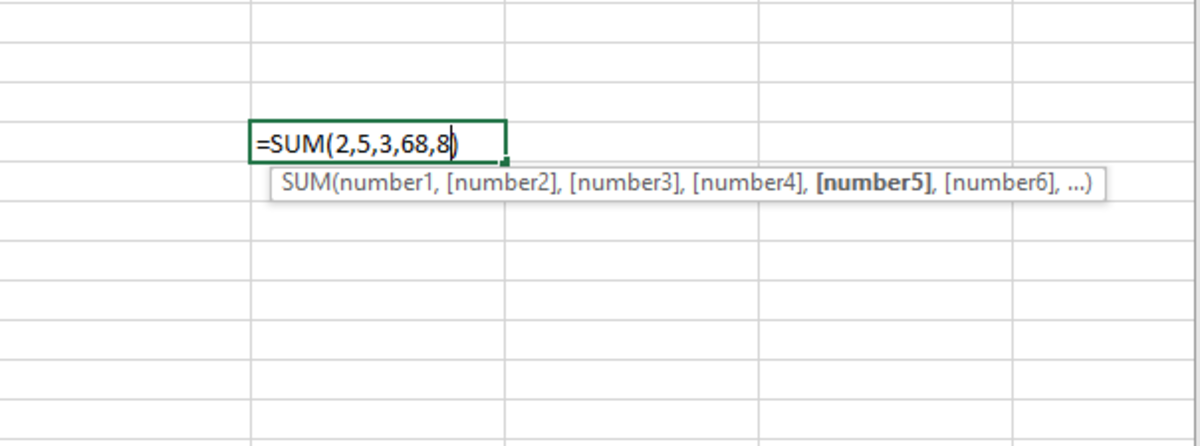In the above illustration, the SUM function is used to sum numbers where the are numbers entered directly into the formula of the function and separated by commas.Created by Joshua Crowder

## SUM Using Named Ranges

Lastly, you may also sum named ranges of numbers as shown in the illustration below. Named range1 outlined in blue is being added to range2 outlined in red.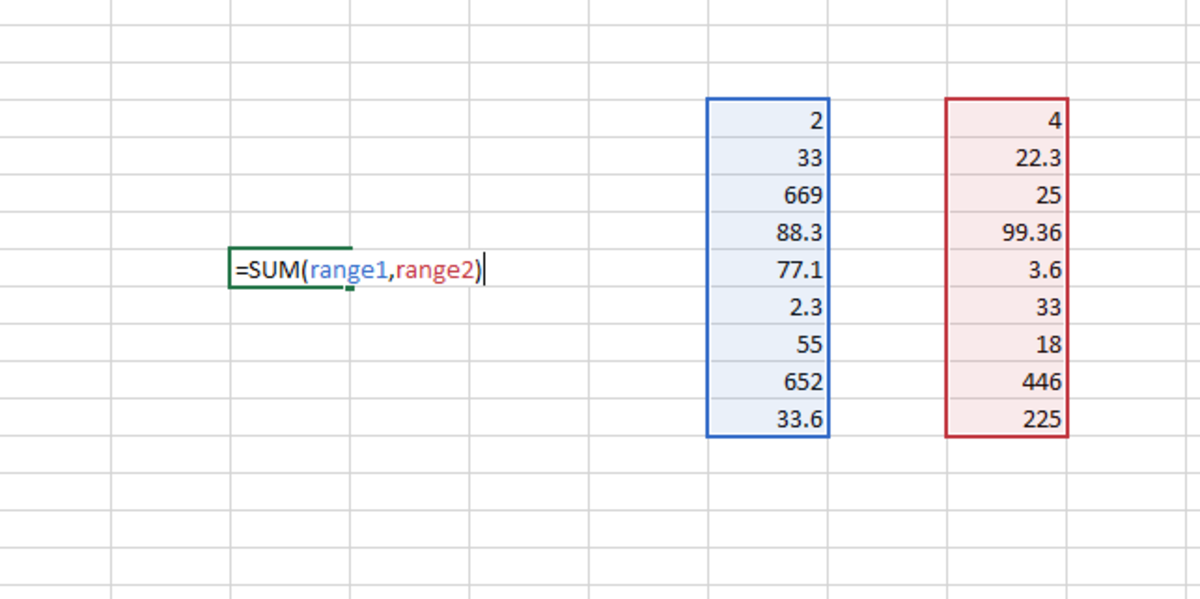In the above illustration, the SUM function is used to sum named ranges that are made up of numbers. Range names are used in the formula separated by commas to sum all numbers in the named ranges. Created by Joshua Crowder

It's worth noting that each of the 4 examples described can be used together interchangeably. Ranges can be used and followed by cell references, numbers, and named ranges as long as the correct syntax is used.

## Inserting the SUM Function

The SUM function can be inserted into a cell using two methods. In one method, you search for the function from the function library, and the other involves the use of an insert function button. Each can be completed in a few steps and is described in the following sections.

## Inserting the SUM Funciton

To use this method, a cell is first selected. Next, the formulas tab is selected, and the "Math and Trig" button is be chosen from the function library. Next, SUM can be chosen from the dropdown menu. These steps are shown here in bold and in the illustration below.

Formulas → Math & Trig → SUM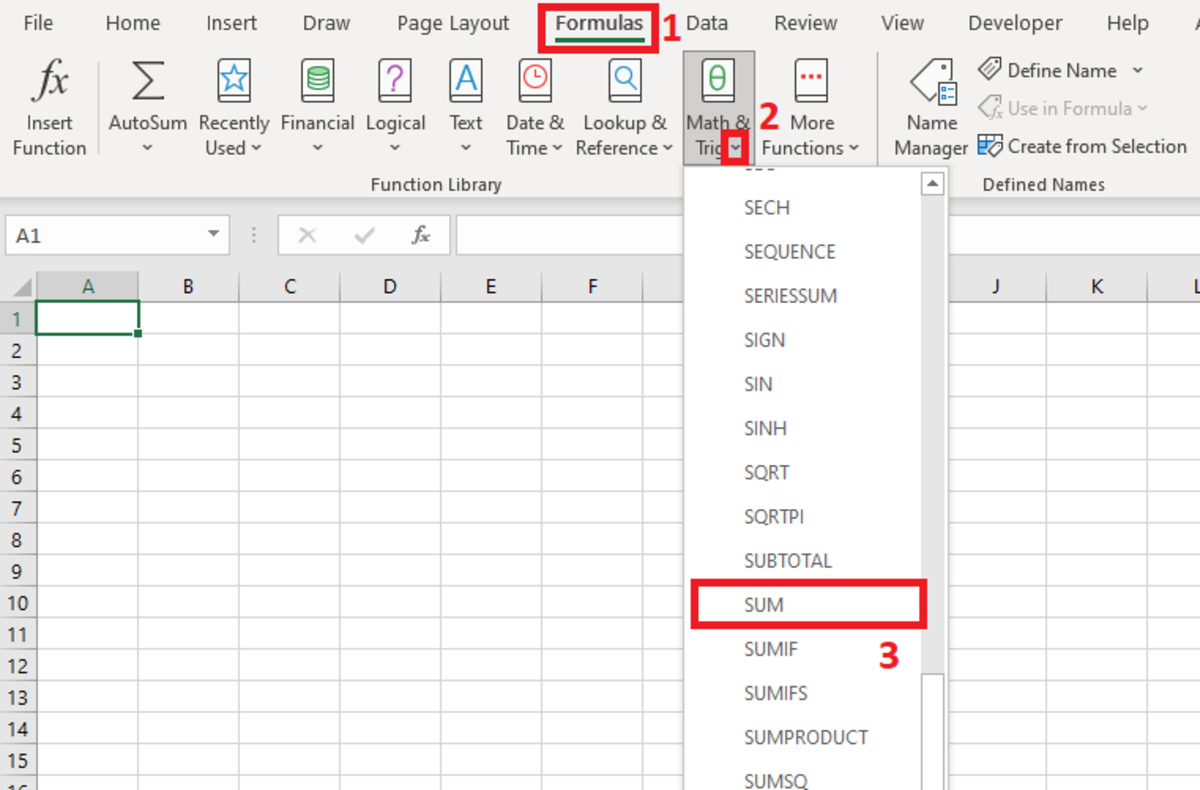The illustration shows how to navigate to insert the SUM function from the formulas tab. This can be accomplished by completing the following steps: Formulas→Math & Trig→SUMCreated by Joshua Crowder

After the functional arguments window appears, you have the option to add as many as 255 numbers, cell references, or ranges that contain 255 cells.

Each time you enter a number or reference into a field, a new one will appear for the next data entry. Once you have added the numbers and/or references that you need, click on the OK button. The functional arguments window can be seen in the example illustration below.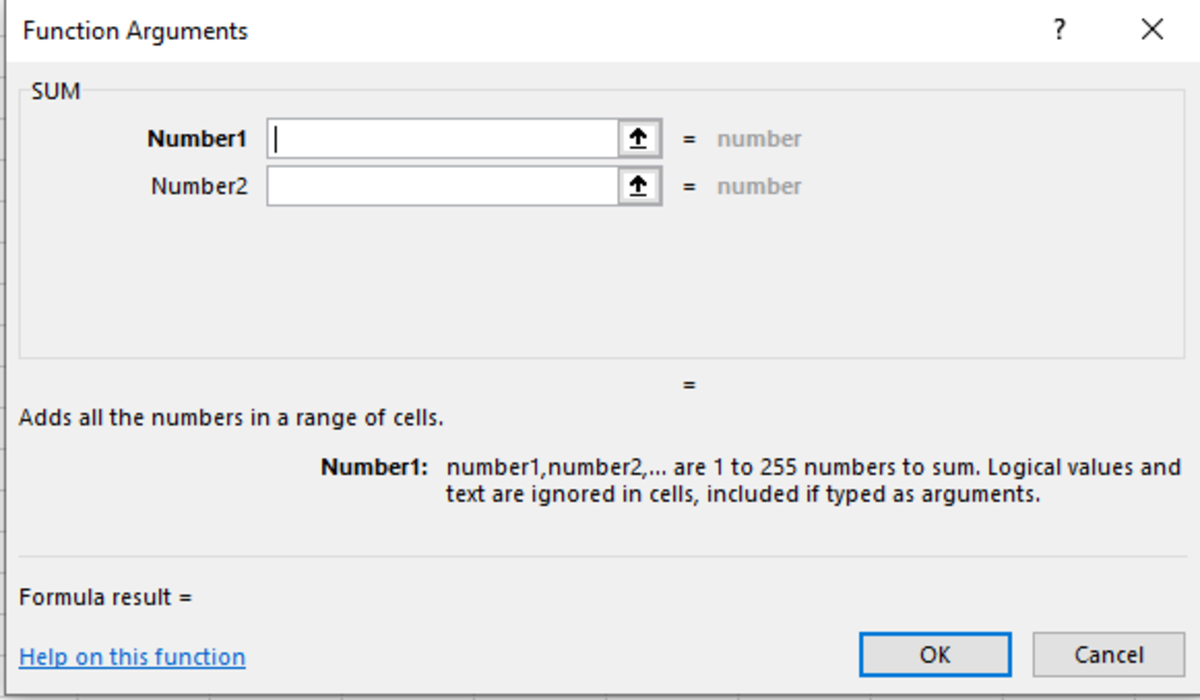In the above functional arguments window, you can add up to 255 different numbers until a memory constant keeps you from adding more. Created by Joshua Crowder

## Inserting From the Insert Function Button

Another way to insert this function would be to insert it from the insert function button. This button is also found under the formulas tab and located on the upper left side of the Excel ribbon in the function library.

Formula Tab → Insert Function

## Inserting the SUM Function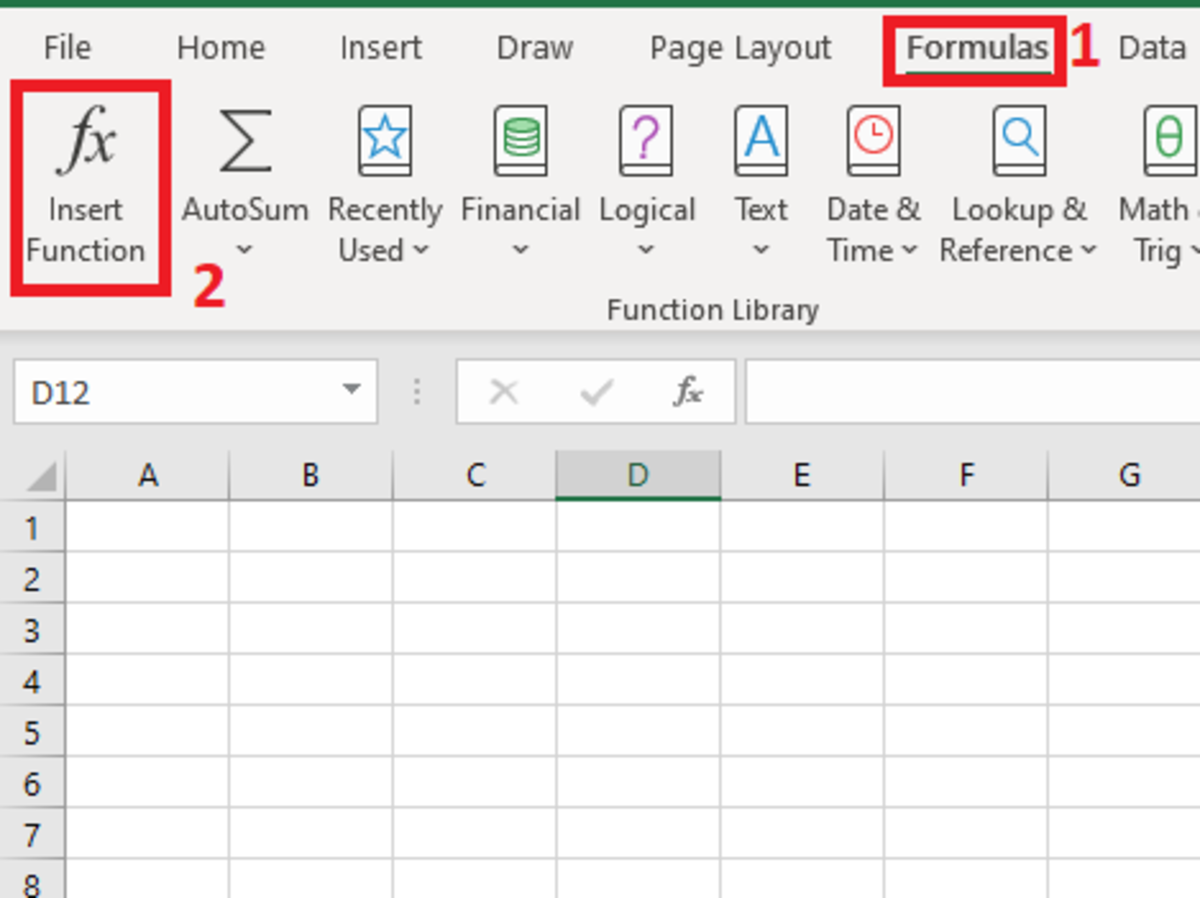The above illustration shows where to find the insert function button on the Excel ribbon found under the formulas tab.

After clicking on the insert function button, the insert function window appears. Text can be entered to search for the function that you are looking for. After clicking OK, you will be directed to the functional arguments window.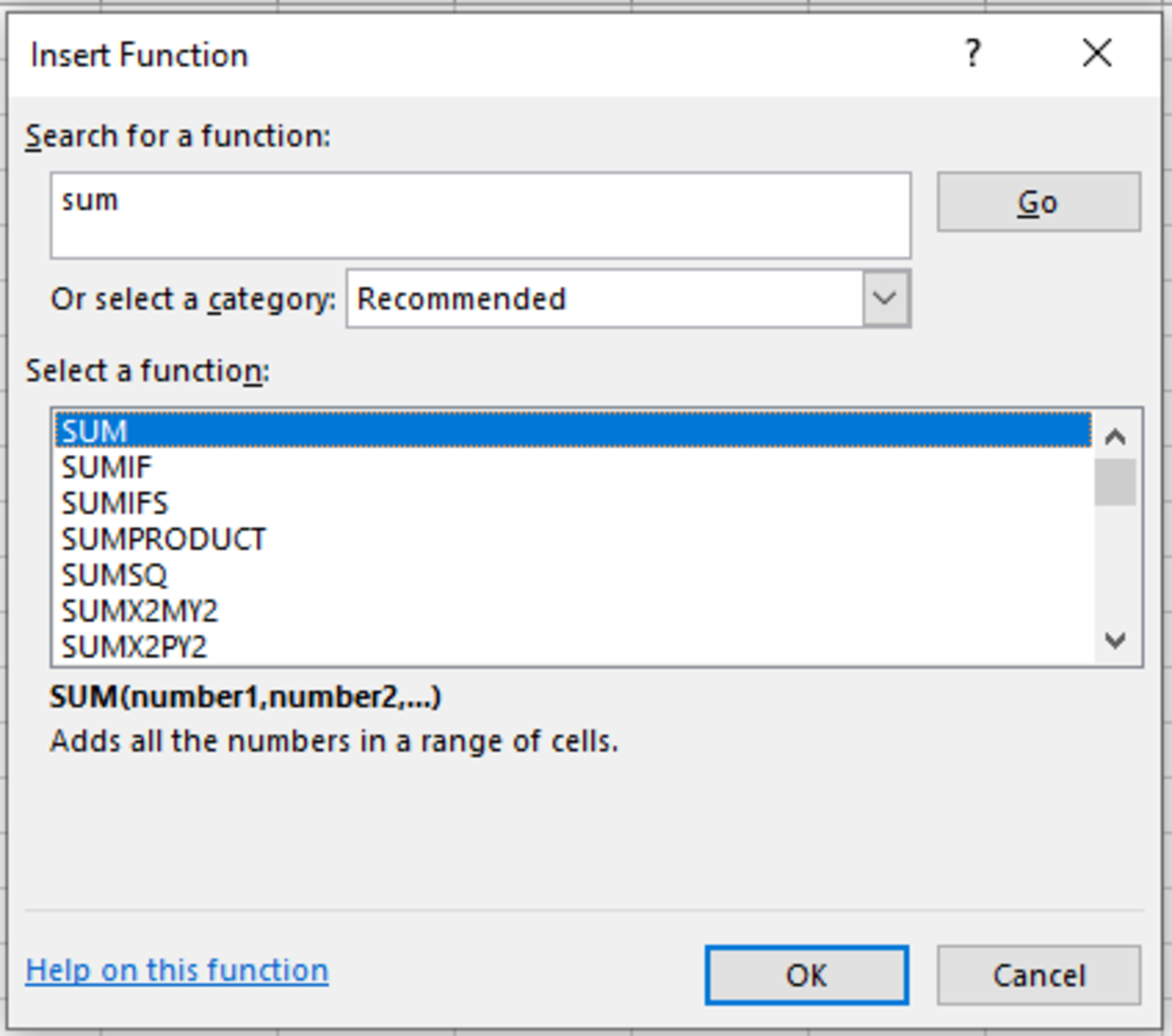The above illustration shows the insert function window where functions to be inserted can be searched for. Created by Joshua Crowder

## Using the SUM Function Shortcut

There is an easy way to bring up the SUM function when you need it. Instead of typing out the formula or inserting the function, you can use a three-stroke shortcut. By holding alt + = you can get the function to appear after you have clicked in a cell. The option will not help you fill the formula like the last two insert options, but it will save you some keystrokes when entering the function manually.

Microsoft. (n.d.). SUM function. Retrieved January 5, 2020, from https://support.office.com/en-us/article/sum-function-043e1c7d-7726-4e80-8f32-07b23e057f89.